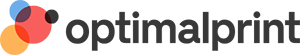Optimalprint
8.6" 10" 14258
8.6/10 (14258 reviews)

# Confirmation Thank You Cards

Categories: See more
Colour
No. of Photos
Format
Orientation
Size
258 designs Sort by:
Square (5.25" x 5.25") - Folded
Small (4.25" x 5.5") - Folded
Standard (7" x 5") - Folded
Small (4.25" x 5.5") - Folded
Standard (5" x 7") - Folded
Standard (5" x 7") - Folded
Small (5.5" x 4.25") - Folded
Small (5.5" x 4.25") - Folded
Small (5.5" x 4.25") - Folded
Small (5.5" x 4.25") - Folded
Small (4.25" x 5.5") - Folded
Small (4.25" x 5.5") - Folded
Square (5.25" x 5.25") - Folded
Small (4.25" x 5.5") - Folded
Small (4.25" x 5.5") - Folded
Square (5.25" x 5.25") - Folded
Square (5.25" x 5.25") - Folded
Small (5.5" x 4.25") - Folded
Small (4.25" x 5.5") - Folded
Small (4.25" x 5.5") - Folded
Small (5.5" x 4.25") - Folded
Small (4.25" x 5.5") - Folded
Small (5.5" x 4.25") - Folded
Small (4.25" x 5.5") - Folded
Small (4.25" x 5.5") - Folded
Standard (5" x 7") - Folded
Small (4.25" x 5.5") - Folded
Small (4.25" x 5.5") - Folded
Square (5.25" x 5.25") - Folded
Small (4.25" x 5.5") - Folded
Small (4.25" x 5.5") - Folded
Small (5.5" x 4.25") - Folded
Standard (7" x 5") - Folded
Standard (7" x 5") - Folded
Small (4.25" x 5.5") - Folded
Small (5.5" x 4.25") - Folded
Small (4.25" x 5.5") - Folded
Square (5.25" x 5.25") - Folded
Small (4.25" x 5.5") - Folded
Small (4.25" x 5.5") - Folded
Small (5.5" x 4.25") - Folded
Small (4.25" x 5.5") - Folded
Square (5.25" x 5.25") - Folded
Small (5.5" x 4.25") - Folded
Small (4.25" x 5.5") - Folded
Square (5.25" x 5.25") - Folded
Small (4.25" x 5.5") - Folded
Square (5.25" x 5.25") - Folded